Appendix A1: Solution of Equation 00-07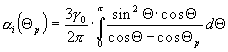(Equ. 00-07)

The integration is transformed into a set of terms, which can be solved seperately. An integratable solution was first presented by Dr. Martin Maurer3, Munich, in 1995 and confirmed by the solution of Dr. Karl Nickel, Freiburg i.Br., 1998. The solution of Dr. Maurer is basis for the here described calculation: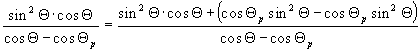(Equ. A1-01a)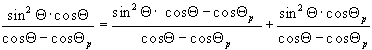(Equ. A1-01b)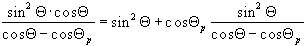(Equ. A1-01c)

The following transformations can be used: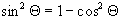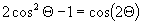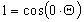(Equ. A1-02)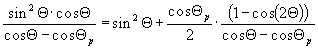(Equ. A1-03a)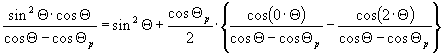(Equ. A1-03b)

This leads to a new formula for the integration (Equ. 00-07):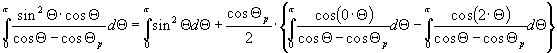(Equ. A1-04)

Solutions for the integrations are given in Multhopp, respectvely Bronstein4: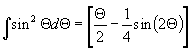(Bro. 275)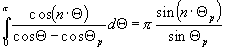(Mul. 09)

Equation Equ. A1-04 now gives: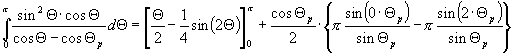(Equ. A1-05)

and from this: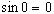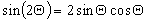(Equ. A1 06)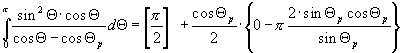(Equ. A1-07a)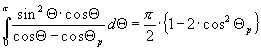(Equ. A1-07b)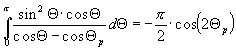(Equ. A1-07c)

This gives the required solution, which can be now written as equation Equ. 00-08: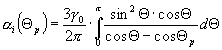(Equ. 00-07)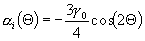or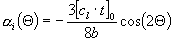(Equ. 00-08)